# Tutorial: Illumination Correction

## Introduction

The goal of illumination correction is to remove uneven illumination of the image caused by sensor defaults (eg., vignetting), non uniform illumination of the scene, or orientation of the objects surface.

Illumination correction is based on background subtraction. This type of correction assumes the scene is composed of an homogeneous background and relatively small objects brighter or darker than the background. There are two major types of background subtraction techniques depending on whether the illumination model of the images can be given as additional images or not:

Note: the results presented in this section are just meant to illustrate the effect of the various illumination correction techniques. The visual quality of this results, in terms of contrast or brightness, can be improved afterwards by using some image enhancement techniques.

## I. Prospective correction

Prospective correction uses additional images obtained at the time of image capture. Two types of additional images can be used:

• A dark image is an image of the scene background acquired with no light.
• A bright image is an image of the scene background acquired with light but without objects.
Note: It is always advantageous to capture several occurrences of bright images and dark images in order to remove noise and attenuate lighting defects. The model of each type of image is then the average of these images:
```dark = ∑ darki
bright = ∑ brighti
```

### 1. Correction from a Dark Image and a Bright Image

The corrected image g(x,y) is obtained using the following transformation:

```            f(x,y) - d(x,y)
g(x,y) = --------------- .C
b(x,y) - d(x,y)
```

where f(x,y) is the original image, d(x,y) is the dark image, b(x,y) is the bright image, and C is a normalization constant used to recover the original colors:

```                                  1
C = mean(f(x,y)) . ----------------------
f(x,y) - d(x,y)
mean( --------------- )
b(x,y) - d(x,y)

```

where mean(i(x,y)) is the mean value of the image i(x,y).

#### Pandore Script (bash)

```psub serous.pan dark.pan tmp1.pan
psub bright.pan dark.pan tmp2.pan
pdiv tmp1.pan tmp2.pan tmp3.pan
pmeanvalue tmp3.pan c1.pan
pdivval c1.pan tmp3.pan tmp4.pan
pmeanvalue serous.pan c2.pan
pmultval c2.pan tmp4.pan output.pan
```

#### Results

The images in the second row are the profiles of the row 132, where the gray level values are represented as vertical bars. We notice that in the input image the illumination is higher on the right side than on the left side. This is corrected in the result image.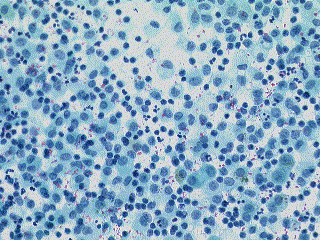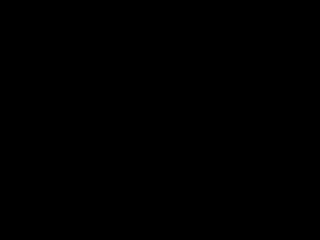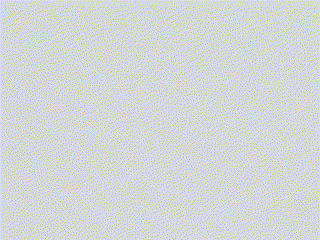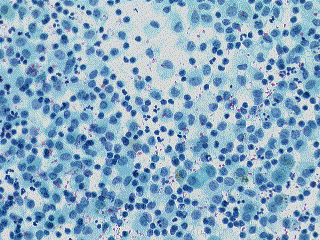Input image. Dark image. Bright image. Corrected output image.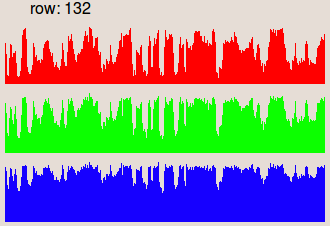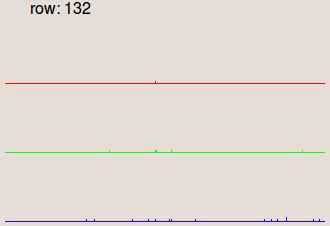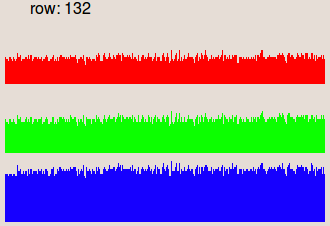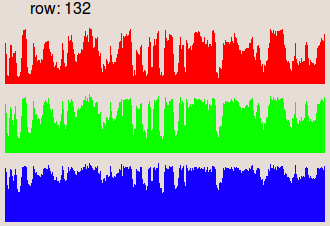Profile of the row 132. Profile of the row 132. Profile of the row 132. Profile of the row 132.

### 2. Correction from a Bright Image

If only the bright image is available, the method uses division of the source image by the bright image if the image acquisition device is linear, or the subtraction of the source image with the bright if the acquisition device is logarithmic with a gamma of 1.

In case of a linear acquisition device, the corrected image g(x,y) is obtained using the following transformation:

```            f(x,y)
g(x,y) = ------ . C
b(x,y)
```

where f(x,y) is the original image, b(x,y) is the bright image, and C is a normalization constant that is used to recover the initial colors:

```                           1
C = mean(f(x,y)) . ------------
f(x,y)
mean( ------ )
b(x,y)
```

where mean(i(x,y)) is the mean value of the image i(x,y).

#### Pandore Script (bash)

```pdiv grain.pan grain_background.pan tmp1.pan
pmeanvalue grain.pan c1.col
pmultval c1.col tmp1.pan tmp2.pan
pmeanvalue tmp1.pan c2.col
pdivval c2.col tmp2.pan grain_out.pan
```

#### Results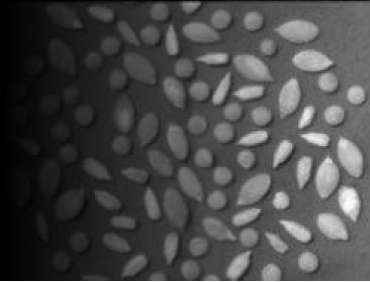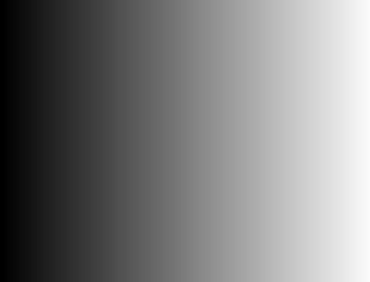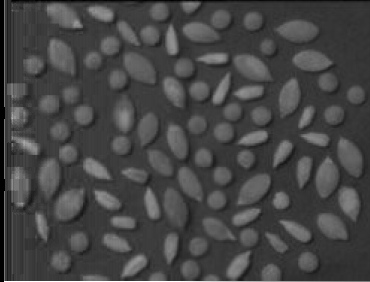Input image. Background image. Output image.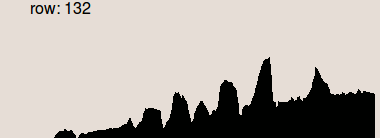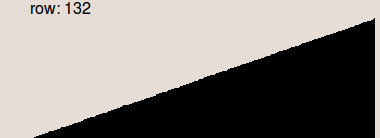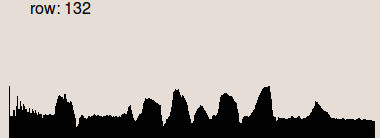Profile of the row 132. Profile of the row 132. Profile of the row 132.

### 3. Correction from a Dark Image

If only the dark image is available, the method consists in subtraction of the dark image with the original image. The corrected image g(x,y) is then obtained using the following transformation:

```   g(x,y) = f(x,y) - d(x,y) + mean(d(x,y))
```

where f(x,y) is the original image, d(x,y) is the dark image and mean(d(x,y)) is the mean value of the dark image.

#### Pandore Script (bash)

```psub serous.pan dark.pan tmp1.pan
pmeanvalue dark.pan c.col
```

#### Results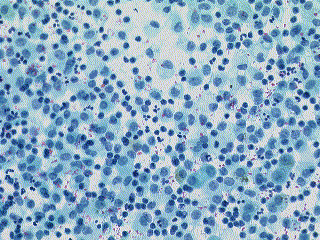Input image. Dark image. Output image.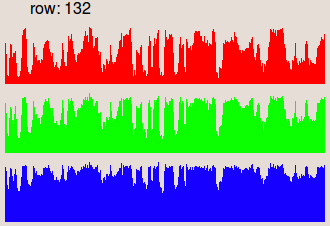Profile of the row 132. Profile of the row 132. Profile of the row 132.

## II. Retrospective correction

When additional image are not available, an ideal illumination model has to be estimated to define the bright image. Therefore, retrospective correction can use the same background subtraction method than the prospective correction with the estimated bright image.

There are different algorithms for estimating the bright image. All of them assume the scene background corresponds to the low frequencies and the objects to the high frequencies. The retrospective correction techniques consist in removing the objects from the background to build the bright image, and then apply the same techniques as the prospective correction.

### 1. Retrospective Correction using Low-pass Filtering

The background is estimated by using a low-pass filtering with a very large kernel. The background is then subtracted from the input image to compensate the illumination.
The corrected image g(x,y) is obtained from the input image f(x,y) by:

```   g(x,y) = f(x,y) - LPF(f(x,y)) + mean(LPF(f(x,y)))
```

where LPF(f(x,y)) is the low-pass filtering of image f(x,y), and mean(LPF(f(x,y))) is the mean value of the low pass image.

The following example uses the Gaussian filter with a kernel of size 20, greater than the size of the characters.

#### Pandore Script (bash)

```pgaussianfiltering 20 page.pan mask.pan
```

#### Results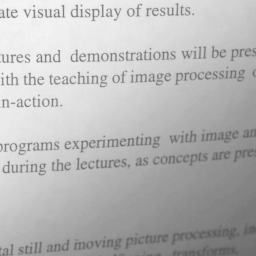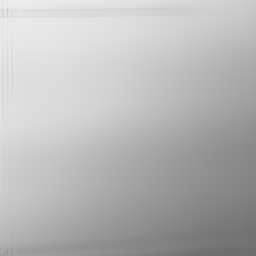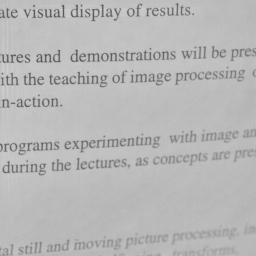Input image. Estimated background image. Output image.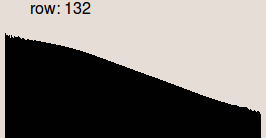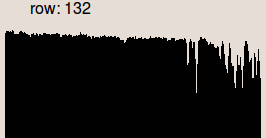Profile of the row 132. Profile of the row 132. Profile of the row 132.

### 2. Retrospective Correction using Homomorphic Filtering

The background is estimated by using homomorphic filtering with a low-pass filter. Homomorphic principle is to remove high frequencies (considered as reflectance) and keep the low frequencies (considered as illumination). The background is removed by highpass filtering the logarithm of the image and then taking the exponent (inverse logarithm) to restore the image.

The corrected image g(x,y) is obtained from the input image f(x,y) by:

```   g(x,y) = exp(LPF(log(f(x,y)))) . C
```

where LPF(f(x,y)) is the low-pass filter of f(x,y) and C is the normalization coefficient given by:

```              mean(f(x,y))
C = ----------------------------
f(x,y)
mean( --------------------- )
exp(LPF(log(f(x,y))))
```

where mean(i(x,y)) is the mean value of the image i(x,y).

The following example uses the Butterworth filter with a cutoff value of 3.

Note that this technique does not really yield accurate results.

#### Pandore Script (bash)

```cutin=0
# the less the cutoff value, the stronger filtering.
cutoff=3
order=2

homomorphicfiltering(){
paddcst 1 \$1 i0.pan # The zero log problem
plog i0.pan i0.pan
psetcst 0 i0.pan i1.pan
pfft i0.pan i1.pan i2.pan i3.pan
pproperty 0 i2.pan
ncol2=`pstatus`
pproperty 1 i2.pan
nrow2=`pstatus`
pproperty 2 i2.pan
ndep2=`pstatus`
pbutterworthfilter \$ncol2 \$nrow2 \$ndep2 0 \$cutin \$cutoff \$order i4.pan
pmult i2.pan i4.pan i5.pan
pmult i3.pan i4.pan i6.pan
pifft i5.pan i6.pan i7.pan i8.pan

pproperty 0 \$1
ncol1=`pstatus`
pproperty 1 \$1
nrow1=`pstatus`
pproperty 2 \$1
ndep1=`pstatus`

pextractsubimage 0 0 0 \$ncol1 \$nrow1 \$ndep1 i7.pan i9.pan
pexp i9.pan i11.pan

pclipvalues 0 255 i12.pan  i13.pan
pim2uc i13.pan \$2
}

multiplicative-correction() {
pdiv \$1 \$2 i14.pan
pmeanvalue \$1 col.pan; c1=`pstatus`
pmeanvalue i14.pan col.pan; c2=`pstatus`
c=`echo "scale = 1; \$c1/\$c2" | bc`

pmultcst \$c i14.pan \$3
}

pproperty 3 page.pan; bands=`pstatus`
if [ \$bands -eq 1 ]
then
# grayscale image
else
# color image
pimc2img 0 input1.pan r.pan
pimc2img 1 input1.pan g.pan
pimc2img 2 input1.pan b.pan
pimg2imc 0 outr.pan outg.pan outb.pan out.pan
fi
```

#### Results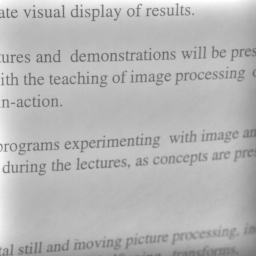Input image. Estimated background image. Output image.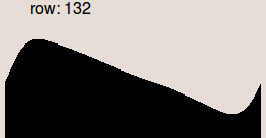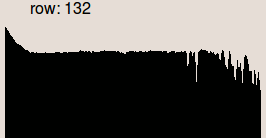Profile of the row 132. Profile of the row 132. Profile of the row 132.

### 3. Retrospective Correction using Morphological Filtering

The background is estimated by mathematical morphology opening or closing. The estimated background is then subtracted from the original image. The total sequence of operations corresponds to a top hat of the image. Top hat removes high frequencies (considered as reflectance) and keeps low frequencies (considered as illumination). Black top hat is used for clear background and white top hat is used for dark background.

If the background is clear, the corrected image g(x,y) is obtained using:

```   g(x,y) = BTH[f(x,y)] + mean(closing(f(x,y)))
g(x,y) = [f(x,y) - closing(f(x,y))] + mean(closing(f(x,y)))
```

where mean(closing(f(x,y))) is the mean value of the closed image.

The following example uses a disc structuring element with size 11, greater than the size of the objects (characters).

#### Pandore Script (bash)

```# black top hat
pdilatation 2 5 page.pan b.pan
# normalization
```

#### Results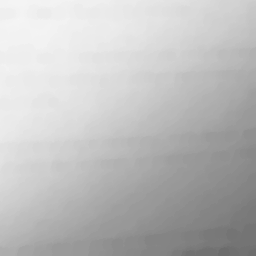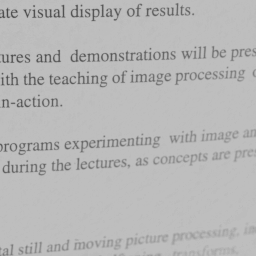Input image. Estimated background image. Output image.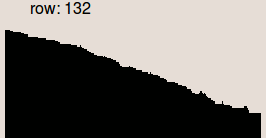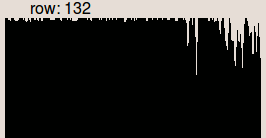Profile of the row 132. Profile of the row 132. Profile of the row 132.

### 4. Retrospective Correction using Linear Regression

The background is estimated by using orthogonal linear regression. The background is approximated by a plane, so it is not suitable for complex background.

The corrected image g(x,y) is obtained from the input image f(x,y) by:

```   g(x,y) = (f(x,y) - LR(f(x,y)) - mean(LR(f(x,y)))
```

where LR(f(x,y)) is the linear regression of f(x,y) in x and y, and mean(LR(f(x,y))) is the mean value of the linear regression image.

#### Pandore Script (bash)

```plinearregression page.pan mask4.pan
```

#### Results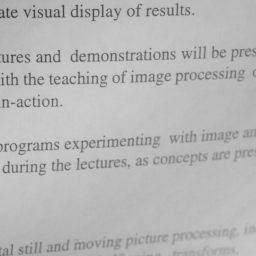Input image. Estimated background image. Output image.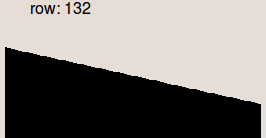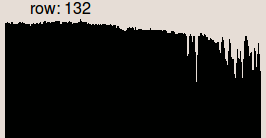Profile of the row 132. Profile of the row 132. Profile of the row 132.

### 5. Retrospective Correction Based on Surface Fitting with polynomial

The background is estimated from a least square fitting of a polynomial that approximates the background. The background is then subtracted from the input image to compensate the illumination. The corrected image g(x,y) is obtained from the input image f(x,y) by:

```   g(x,y) = (f(x,y) - b(f(x,y))) - mean(b(f(x,y)))
```

where b(f(x,y)) is a polynomial that approximates the background, and mean(b(f(x,y))) is the mean value of the polynomial image.

In the following examples, two types of polynomials has been used:

• Second-order polynomial
• Legendre polynomial.

#### Second-Order Polynomial

The first following example uses second-order polynomial. The operator needs a mask to select only background pixels. The mask defines the list of pixels that can be used to compute the polynomial approximation.

The order of the polynomial can be selected separately for x, y, and mixed terms. For example, with orders 2, 3, and 2 for x, y, and xy respectively, the polynomial will be:

```  a+b*x+c*x^2+d*y+e*y^2+f*y^3+g*xy
```

#### Pandore Script (bash)

```ppolynomialfitting 2 2 1 page.pan page_mask.pan mask5.pan
```

#### Results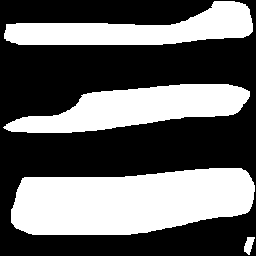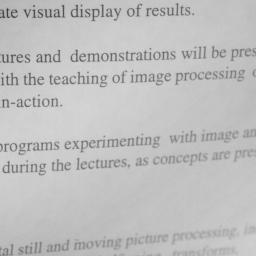Input image. Mask image. Estimated background image. Output image..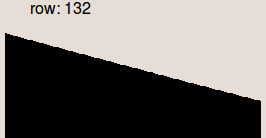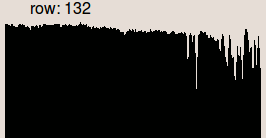Profile of the row 132. . Profile of the row 132. Profile of the row 132.

#### Legendre Polynomial

The second following example uses Legendre polynomial. This technique uses the orthogonality relation of the Legendre polynomials to expand an image as a double sum of those functions. The sum is then evaluated to produce an image that approximates a projection onto the space of polynomial images.

#### Pandore Script (bash)

```plegendrepolynomialfitting 2 2 page.pan mask6.pan
```

#### ResultsInput image. Estimated background image. Output image.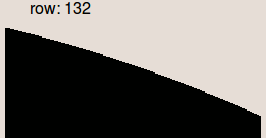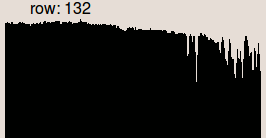Profile of the row 132. Profile of the row 132. Profile of the row 132.

### 6. Retrospective Correction for Color Images

The previous methods cannot be applied per se to color images because they used channel-wise processing and thus alter the spectral composition of colors (creation of new colors).

The solution is to convert the image into the HSL color space and applied the correction to the lightness channel.

The example provided below uses the morphological approach of the correction.

#### Pandore Script (bash)

```prgb2hsl whiteboard.pan data1.pan
pgetband 0 data1.pan data44.pan
pgetband 1 data1.pan data45.pan
pgetband 2 data1.pan data36.pan
pdilatation 1 5 data36.pan data37.pan
perosion 1 5 data37.pan data38.pan
pmeanvalue data38.pan data41.pan
psub data36.pan data38.pan data40.pan
pimg2imc 4 data44.pan data45.pan data42.pan data43.pan
phsl2rgb data43.pan out7.pan
```

#### Results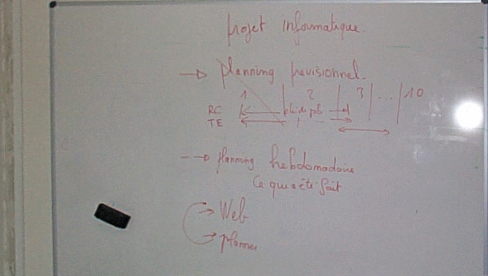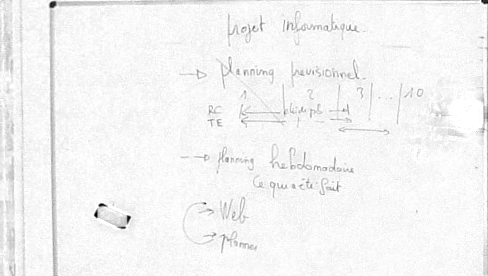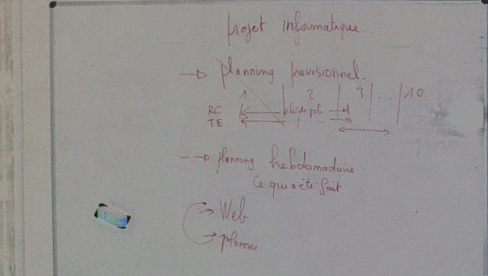Input image. Estimated background image. Output image.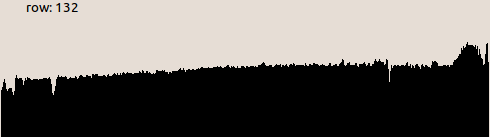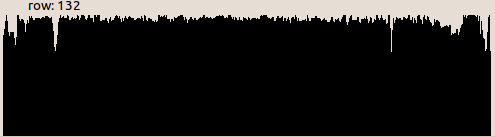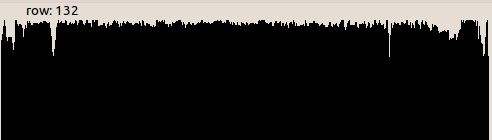Profile of the row 132. Profile of the row 132. Profile of the row 132.

## Reference

John C. Russ, "The image processing handbook, Fifth edition", CRC Press, 2006.

The Pantheon project
Image Team GREYC Laboratory
UMR CNRS 6072 - ENSICAEN - University of Caen, France